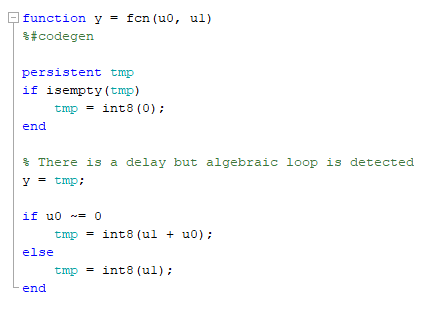## Using Persistent Variables and fi Objects Inside MATLAB Function Blocks for HDL Code Generation

These guidelines illustrate the recommended settings when using persistent variables inside MATLAB Function blocks in your model. The MATLAB Function block is available in the User-Defined Functions block library. A persistent variable in a MATLAB Function block acts similar to a delay element in your Simulink® model.

Each guideline has a severity level that indicates the level of compliance requirements. To learn more, see HDL Modeling Guidelines Severity Levels.

### Update Persistent Variables at End of MATLAB Function

`2.8.1`

#### Severity

Strongly Recommended

#### Description

To make sure that the persistent variables inside the MATLAB Function block map to a register on the target FPGA device, update the persistent variable at the end of the MATLAB® code inside the MATLAB Function block. Do not update the persistent variable before its value is read or used by the function.

For example, this MATLAB code is not recommended because the function updates the persistent variable `FF0` is updated before the value is read at the output.

```function FF_out0 = fcn(FF_in) %#codegen persistent FF0 if isempty(FF0) FF0 = zeros(1, 'like', FF_in); end % Incorrect order of FF update FF0 = FF_in % Output FF0. FF_out0 is NOT delayed FF_out0 = FF0; ```

This MATLAB code is recommended because the value is written to `FF0` at the end of the code.

```function FF_out0 = fcn(FF_in) %#codegen persistent FF0 if isempty(FF0) FF0 = zeros(1, 'like', FF_in); end % Output FF0 FF_out0 = FF0; % Write FF update at the end of the code FF0 = FF_in ```

### Avoid Algebraic Loop Errors from Persistent Variables inside MATLAB Function Blocks

Guideline ID

`2.8.2`

Severity

Mandatory

Description

When your Simulink® model contains MATLAB Function blocks inside a feedback loop and uses persistent variables, compiling or simulating the model might generate algebraic loop errors. To simulate the model and generate HDL code, use nondirect feedthrough.

In certain cases, the persistent delay in the MATLAB Function block inside a feedback loop causes an algebraic loop error. When you use direct feedthrough, the output of the block directly depends on the input. When Allow direct feedthrough is cleared, the output of the block depends on the internal state and properties and does not depend on the input. The nondirect feedthrough semantics prevents algebraic loops errors by making the outputs depend only on the state.

For an example, open the model `hdlcoder_MLFB_avoid_algebraic_loops`.

```modelname = 'hdlcoder_MLFB_avoid_algebraic_loops'; blkname = 'hdlcoder_MLFB_avoid_algebraic_loops/Subsystem_AlgLoop_Issue/MATLAB Function1'; open_system(modelname) ```When you simulate the model, the algebraic loop error message is displayed. The MATLAB Function block `hdlcoder_MLFB_avoid_algebraic_loops/Subsystem_AlgLoop_Issue/MATLAB Function` uses a persistent variable inside a MATLAB Function block.

```open_system(blkname) ```To avoid this error, use nondirect feedthrough. To specify nondirect feedthrough at the command line, create a `MATLABFunctionConfiguration` object by using `get_param` function, and then change the property value `AllowDirectFeedthrough`:

```MLFBConfig = get_param(blkname, 'MATLABFunctionConfiguration'); MLFBConfig.AllowDirectFeedthrough = 0; ```

To specify nondirect feedthrough from the UI:

1. Open the MATLAB Function block `MATLAB Function1`.

2. Open the Property Inspector. In the Modeling tab, in the Design section, click Property Inspector.

3. In the Property Inspector, open the Advanced section and clear the Allow direct feedthrough check box.

The model now simulates without algebraic errors. You can now generate HDL code for the Subsystem block `Subsystem_AlgLoop_Issue`.

```open_system(modelname) set_param('hdlcoder_MLFB_avoid_algebraic_loops', 'SimulationCommand', 'Update') makehdl('hdlcoder_MLFB_avoid_algebraic_loops/Subsystem_AlgLoop_Issue') ```

### Use hdlfimath Setting and Specify fi Objects inside MATLAB Function Block

`2.8.3`

#### Severity

Strongly Recommended

#### Description

`fimath` properties define the rules for performing arithmetic operations on fi objects. To specify `fimath` properties that govern arithmetic operations, use a `fimath` object. To see the default `fimath` property settings, run this command:

`F = fimath`
```F = RoundingMethod: Nearest OverflowAction: Saturate ProductMode: FullPrecision SumMode: FullPrecision```

The default `fimath` settings reduce rounding errors and overflows. However, HDL code generation for a MATLAB Function block that uses these settings can incur additional resource usage on the target FPGA device. To avoid the additional logic, use `hdlfimath`. The `hdlfimath` function is a utility that defines `fimath` properties optimized for HDL code generation. To see the default `hdlfimath` settings, run this command:

`H = hdlfimath`
```H = RoundingMethod: Floor OverflowAction: Wrap ProductMode: FullPrecision SumMode: FullPrecision```

HDL code generation for a MATLAB Function block that uses these settings avoids the additional resource usage and saves area on the target FPGA device.

To specify these settings for a MATLAB Function block:

• Double-click the MATLAB Function block.

• In the Modeling tab, in the Design section, click the Property Inspector.

• In the Property Inspector, open the Fixed-point properties section. For:

• Treat these inherited Simulink signal types as fi objects, select ```Fixed-point & Integer```.

If you use the default `Fixed-point` setting, fixed-point data types specified by using fi objects and built-in integer types such as `int8` and `int16` are treated differently. When you use built-in integer types, the output data type for integer type calculations becomes the same as the input data type. The bit width is not expanded to perform numeric calculation.

• MATLAB FUNCTION FIMATH, select Specify Other and then enter `hdlfimath`.

To perform rounding operations that are different from the default hdlfimath settings, specify these settings explicitly by using the fi object as illustrated below.

`A = fi(4.9, 1, 8)`
```A = 4.8750 DataTypeMode: Fixed-point: binary point scaling Signedness: Signed WordLength: 8 FractionLength: 4```

`B = fi(2.3, 1, 10)`
```B = 2.2969 DataTypeMode: Fixed-point: binary point scaling Signedness: Signed WordLength: 10 FractionLength: 7```

`C = fi(A+B, 'RoundingMethod', 'Nearest', 'OverflowAction', 'Saturate') `
```C = 7.1719 DataTypeMode: Fixed-point: binary point scaling Signedness: Signed WordLength: 12 FractionLength: 7 RoundingMethod: Nearest OverflowAction: Saturate ProductMode: FullPrecision SumMode: FullPrecision```

To make sure that the fimath settings are specified according to hdfimath for the MATLAB Function block, you can run the check Check for MATLAB Function block settings.